# AC自动机 算法详解(图解)及模板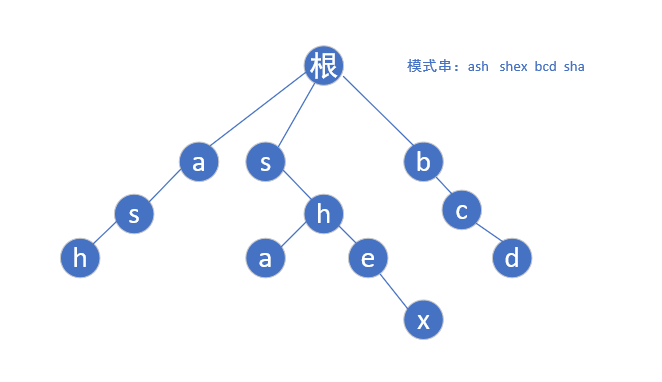ac自动机,就是在tire树的基础上,增加一个fail指针,如果当前点匹配失败,则将指针转移到fail指针指向的地方,这样就不用回溯,而可以路匹配下去了.(当前模式串后缀和fail指针指向的模式串部分前缀相同,如abcebcd,我们找到c发现下一个要找的不是e,就跳到bcd中的c处,看看此处的下一个字符(d)是不是应该找的那一个)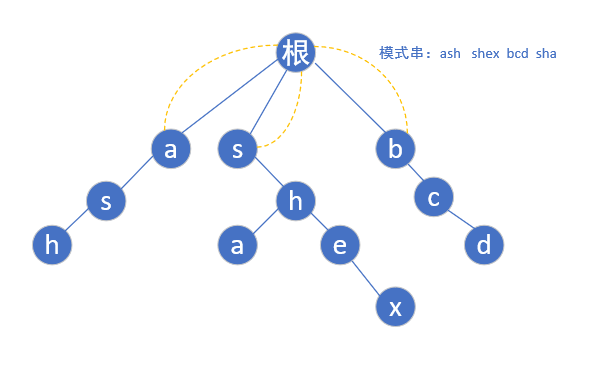(根节点为第0层)第二层a的子节点为s,但是我们还是要从a-z遍历,如果不存在这个子节点我们就让他指向根节点(如下图红色的a)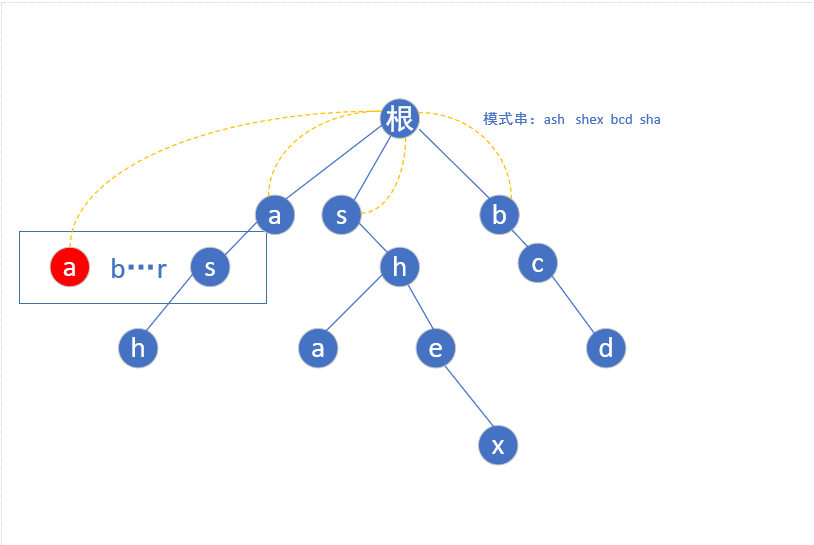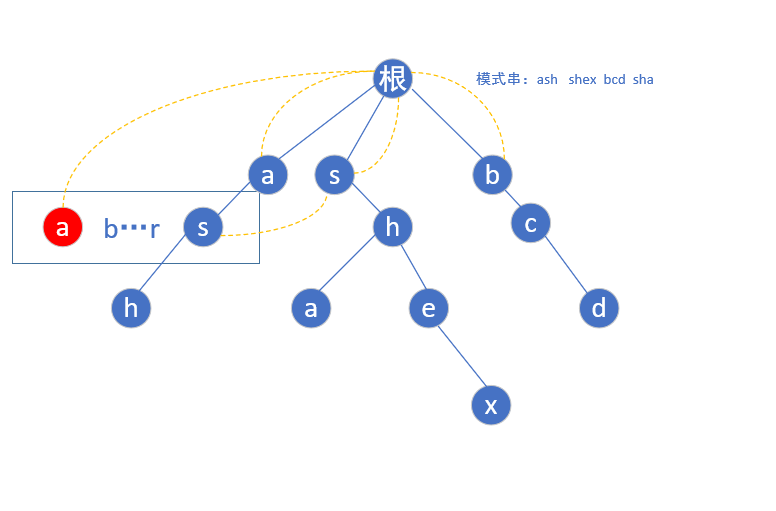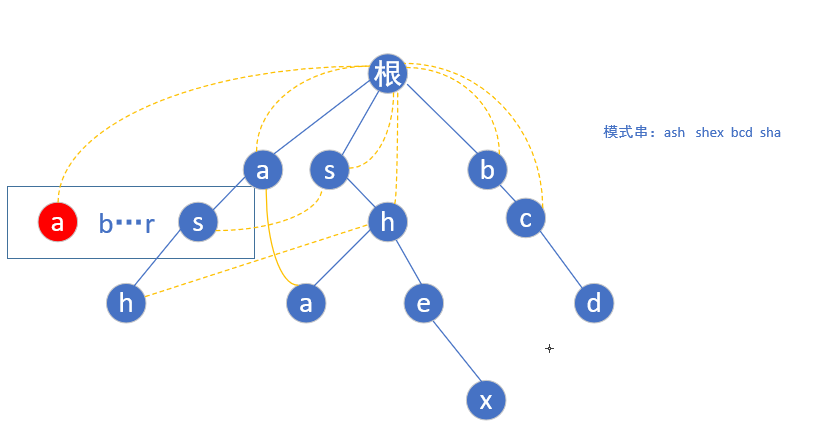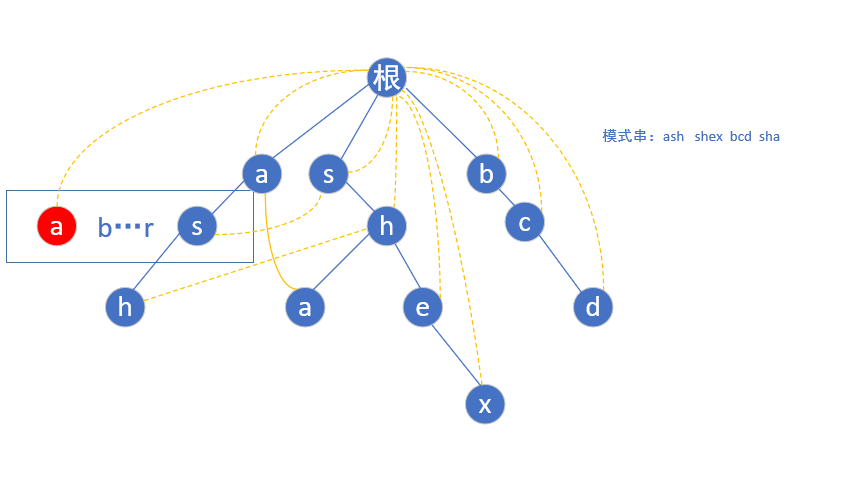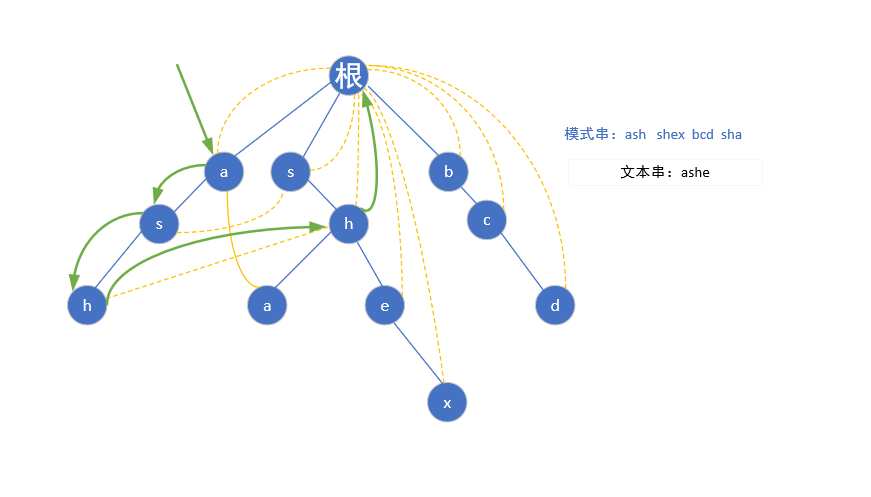#include <queue>
#include <cstdlib>
#include <cmath>
#include <cstdio>
#include <string>
#include <cstring>
#include <iostream>
#include <algorithm>
using namespace std;
typedef long long ll;
const int maxn =  2*1e6+9;

int trie[maxn]; //字典树
int cntword[maxn];  //记录该单词出现次数
int fail[maxn];     //失败时的回溯指针
int cnt = 0;

void insertWords(string s){
int root = 0;
for(int i=0;i<s.size();i++){
int next = s[i] - 'a';
if(!trie[root][next])
trie[root][next] = ++cnt;
root = trie[root][next];
}
cntword[root]++;      //当前节点单词数+1
}
void getFail(){
queue <int>q;
for(int i=0;i<26;i++){      //将第二层所有出现了的字母扔进队列
if(trie[i]){
fail[trie[i]] = 0;
q.push(trie[i]);
}
}

//fail[now]    ->当前节点now的失败指针指向的地方
tire[now][i] -> 下一个字母为i+'a'的节点的下标为tire[now][i]
while(!q.empty()){
int now = q.front();
q.pop();

for(int i=0;i<26;i++){      //查询26个字母
if(trie[now][i]){
//如果有这个子节点为字母i+'a',则
//让这个节点的失败指针指向(((他父亲节点)的失败指针所指向的那个节点)的下一个节点)
//有点绕,为了方便理解特意加了括号

fail[trie[now][i]] = trie[fail[now]][i];
q.push(trie[now][i]);
}
else//否则就让当前节点的这个子节点
//指向当前节点fail指针的这个子节点
trie[now][i] = trie[fail[now]][i];
}
}
}

int query(string s){
int now = 0,ans = 0;
for(int i=0;i<s.size();i++){    //遍历文本串
now = trie[now][s[i]-'a'];  //从s[i]点开始寻找
for(int j=now;j && cntword[j]!=-1;j=fail[j]){
//一直向下寻找,直到匹配失败(失败指针指向根或者当前节点已找过).
ans += cntword[j];
cntword[j] = -1;    //将遍历国后的节点标记,防止重复计算
}
}
return ans;
}

int main() {
int n;
string s;
cin >> n;
for(int i=0;i<n;i++){
cin >> s ;
insertWords(s);
}
fail = 0;
getFail();
cin >> s ;
cout << query(s) << endl;
return 0;
}


03-185992
04-23126205-16693
06-096万+
04-015880
06-162万+
05-085373
01-2955
10-023万+